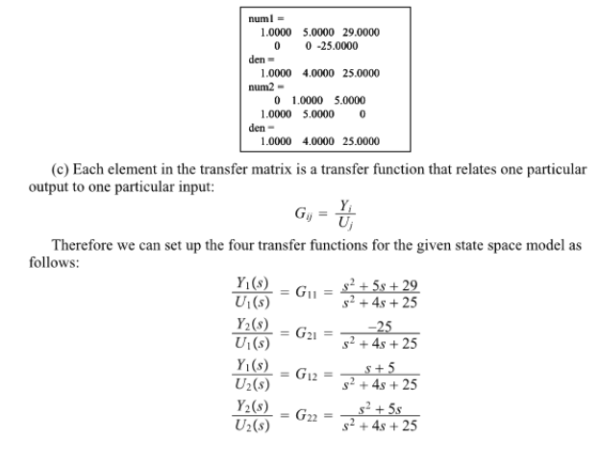Consider the system defined by the following matrix. This system involves two inputs and two outputs. Four transfer functions are involved: Y1(s)/U1(s), Y2(s)/U1(s), Y1(s)/U2(s), and Y2(s)/U2(s). (When considering input u1, we assume that input u2 is zero, and vice versa.) Obtain the transfer matrix (consisting of the preceding four transfer functions of the system. (a) Obtain the transfer matrix G(s) by hand. (b) Verify your answers by using ss2tf in MATLAB. (c) Explicityly write out the four individual transfer functions.Consider the system defined by the following matrix. This system involves two inputs and two outputs. Four transfer functions are involved: Y1(s)/U1(s), Y2(s)/U1(s), Y1(s)/U2(s), and Y2(s)/U2(s). (When considering input u1, we assume that input u2 is zero, and vice versa.) Obtain the transfer matrix (consisting of the preceding four transfer functions of the system. (a) Obtain the transfer matrix G(s) by hand. (b) Verify your answers by using ss2tf in MATLAB. (c) Explicityly write out the four individual transfer functions.

System Dynamics Page 2 dynamics dynamics dynamics dynamics dynamics dynamics dynamics System dynamics Page 3